# Alfvén waves

(diff) ← Older revision | Latest revision (diff) | Newer revision → (diff)

Waves in an ionized fluid, for which the only restoring force is magnetic. This definition allows for different background media (homogeneous or not) under an external magnetic field (which may be uniform, or vary in strength and/or direction), for the presence of dissipation and other effects (displacement, Ohmic or Hall currents, fluid viscosity, mean flow, multiple ion species); it excludes other types of waves (sound, gravity and inertial) associated with restoring forces of non-magnetic origin.

The simplest case [a1] is a perfectly conducting, homogeneous, ionized fluid under a uniform external magnetic field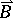. For an Alfvén wave the perturbations of the velocity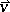and magnetic field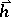are parallel and transverse to the external magnetic field,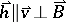, and propagate along magnetic field lines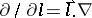with: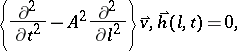(a1)

at the Alfvén speed (a2):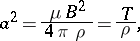(a2)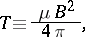whereis the magnetic permeability andthe mass density. Alfvén waves are analogous [a2] to the transverse oscillations of a string, with the velocityand magnetic fieldperturbations replacing the displacement, the magnetic field lines replacing the string, and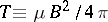"magnetic" tension. Under these simple conditions, the velocity perturbation is the fraction of the Alfvén speed equal to the magnetic field perturbation as a fraction of the external magnetic field: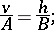(a3)

this implies, using (a2), the equi-partition of kineticand magnetic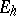energies:(a4)

It also implies that the energy flux is the total energy,(a5)

times the Alfvén speed: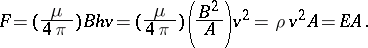(a6)

The energy density and flux satisfy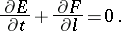(a7)

Alfvén waves, being transversal, are incompressible: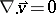(note that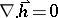always, by the Maxwell equations).

In a compressible fluid, the pressure acts as a restoring force, and one obtains sound waves. The combination is magneto-acoustic waves [a3], [a4], which have three modes: i) unchanged Alfvén mode, because it is incompressible; and sound waves modified into two coupled slow ii) and fast iii) modes. Considering a stratified fluid (e.g., an atmosphere) and adding gravity as a restoring force, one has magneto-acoustic-gravity waves [a2], [a5], [a6], [a7] and Alfvén-gravity waves decouple only if the horizontal wave-vector (which exists only in the direction transverse to stratification) lies in the plane of gravity and the external magnetic field. Adding rotation and the Coriolis force as the fourth restoring force leads to magneto-acoustic-gravity-inertial waves [a2], [a8], for which decoupling of Alfvén-gravity modes is generally not possible. Below, the Alfvén waves are uncoupled to other types of waves in fluids.

Alfvén waves in a stratified medium, e.g., with density a function of altitude, but under a uniform external magnetic field [a9], and isothermal conditions, satisfy different equations [a10] for the velocity and magnetic field perturbations: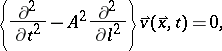(a8)(a9)

where the Alfvén speed is, in general, non-uniform. The relation (a3) no longer holds, and thus equi-partition of energies breaks down (a4), (a5), and the simplification of the energy flux (a6) fails. Examples of a non-constant magnetic field are: i) a radial magnetic field [a10], for which Alfvén waves remain incompressible; and ii) a spiral magnetic field [a12], for which they are not divergence free (compressive Alfvén waves). Alfvén waves have also been considered in non-isothermal atmospheres [a13], [a14], and in magnetic flux tubes [a15].

The Alfvén wave equations are usually deduced from the equations of magneto-hydrodynamics [a16], neglecting the displacement current in comparison with the electric current (cf. also Magneto-hydrodynamics, mathematical problems in). The effect of the displacement current on Alfvén waves has been studied [a17]. Another effect is Hall currents, resulting from the spiralling of electrons around the magnetic field [a18], [a19]. If the external magnetic field is non-uniform, the ion-gyro (or spiralling) frequency varies with altitude, and where it equals the wave frequency a critical layer occurs [a20], [a21]. A critical layer is a singularity of the wave equation, where wave absorption, reflection or transformation can occur. A critical layer also occurs for Alfvén-gravity waves in the presence of Ohmic currents, i.e., electrical resistance [a22]. Since Alfvén waves are transversal, i.e., incompressible, there are no thermal effects (e.g., conduction or radiation), and the other dissipation mechanism is shear viscosity [a23], [a24]. In a homogeneous medium the dissipative Alfvén wave equation reads [a25]:(a10)

whereis the Ohmic andthe viscous diffusivity. If their product is small, the fourth, biharmonic, term can be omitted [a26]. The wave equation is different forand, and more complicated for dissipative Alfvén–gravity waves, since then, in general,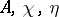are non-uniform [a27], [a28].

Alfvén waves have been observed in the laboratory [a29], and occur in plasma machines and fusion reactors. They may be present in the Earth's molten core, where inertial effects could be important. Alfvén waves have been observed in the solar atmosphere [a30], and could be a mechanism for: i) heating the atmosphere by dissipation [a31], [a32]; or ii) accelerating the solar wind [a33]. Alfvén waves [a34], [a35] propagate with the solar wind to the Earth's ionosphere, and exist in the interplanetary [a15] and probably in the interstellar medium. The case of the solar wind [a33] combines several of the possible influences on Alfvén waves: i) non-uniform background density, decaying towards the Earth; ii) external magnetic field varying in strength and direction along Parker's spiral; iii) background mean flow with velocity exceeding the Alfvén speed, beyond the critical point; iv) presence of multiple ion species. In the distant solar wind, as particle density decreases, Alfvén waves should be considered in the context of plasmas [a36], rather than of magneto-hydrodynamics [a37].

The generation of Alfvén waves results from hydromagnetic turbulence and ionized inhomogeneities [a4], [a38], [a39], e.g., in the photosphere of the sun, where the Alfvén waves propagating in the solar atmosphere and solar wind originate. The solution of the Alfvén wave equation uses:

i) elementary functions, Fourier transforms and dispersion relations for homogeneous media [a1], [a4], [a5], [a6], [a7], [a18], [a20], [a25] (cf. also Fourier transform);

ii) Bessel functions for isothermal atmospheres and other inhomogeneous media [a9], [a10], [a11], [a12], [a13], [a17], [a19], [a30];

iii) hypergeometric functions (cf. also Hypergeometric function) when critical layers are present [a21], [a22], [a23], [a24], [a27], [a28], [a32]. In this case the three singularities represent the initial and asymptotic wave fields, and the wave fields near the critical layer.

Alfvén waves are linear [a37], [a2], even when of finite amplitude, and this is why most of the theory uses linear differential equations with constant or variable coefficients.

How to Cite This Entry:
Alfvén waves. Encyclopedia of Mathematics. URL: http://encyclopediaofmath.org/index.php?title=Alfv%C3%A9n_waves&oldid=14674
This article was adapted from an original article by L.M.B.C. Campos (originator), which appeared in Encyclopedia of Mathematics - ISBN 1402006098. See original article